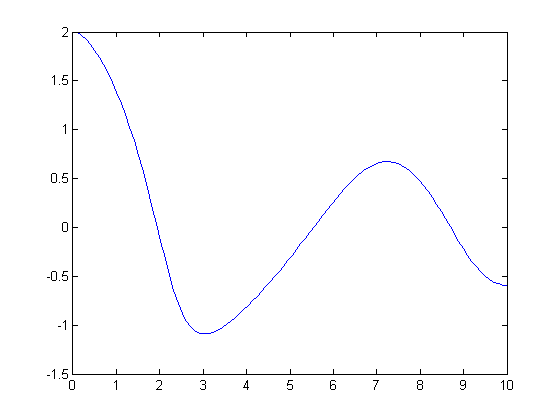## Loren on the Art of MATLABTurn ideas into MATLAB

Note

Loren on the Art of MATLAB has been archived and will not be updated.

# From Symbolic Differential Equations to Their Numeric Solution

Once more, I am pleased to introduce guest blogger Kai Gehrs. Kai has been a Software Engineer at MathWorks for the past five years mainly working on features for the Symbolic Math Toolbox. He has a background in mathematics and computer science and already contributed to my blog in the past.

### Contents

In the article ODEs, from Symbolic to Numeric Code, Loren demonstrated how you can

• Compute a symbolic solution of an ordinary differential equation (ODE) using dsolve
• Convert the symbolic solution to a MATLAB function handle using matlabFunction
• Plot the solution using MATLAB's plot.

In this article, we consider a slightly different scenario. Suppose that we have defined an ODE. First, we try to solve it symbolically. But we find that the symbolic ODE solver cannot find a closed form solution (something which is likely to happen, because only particular classes of ODEs can be solved in closed symbolic form).

Since we cannot solve the ODE symbolically, we must switch to a numeric ODE solver. But numeric solvers require a particular form and type of arguments, which often differ from the arguments of a symbolic solver. Symbolic solvers require a scalar symbolic (= "textbook-like") representation of an ODE. Numeric solvers usually require them to be transformed to a coupled first-order system.

This article demonstrates the following workflow of transforming a symbolic representation of an ODE to a form accepted by the MATLAB numeric ODE solver ode45.

• Define an ODE in a symbolic form using symbolic functions
• Convert it to a coupled first-order system using odeToVectorField
• Create a function handle for the coupled first-order system using matlabFunction
• Solve the differential equation numerically using the MATLAB numeric ODE solver ode45
• Plot the solution using plot.

#### Defining a Differential Equation in Symbolic Form

We start with the second-order non-linear ODE with two given initial values:

$$y'' = (y - y^2) \cdot y' - y, \qquad y = y(t), \qquad y(0) = 2, \qquad y'(0) = 0.$$

We define it in a symbolic form using a symbolic function symfun y(t):

syms y(t);
ode = diff(y,2) == (y-y^2)*diff(y) - y;


When trying to compute a solution of this ODE using dsolve, we see that the symbolic ODE solver does not find an explicit closed form solution:

dsolve(ode)

Warning: Explicit solution could not be found.
ans =
[ empty sym ]


Adding the initial values does not help:

Dy = diff(y); dsolve(ode,y(0) == 2,Dy(0) == 0)

Warning: Explicit solution could not be found.
ans =
[ empty sym ]


So we must use a numeric ODE solver to be able to plot the solution. But before we can convert the symbolic form of the ODE to a function handle accepted by ode45, we must convert the scalar form of the ODE to a coupled first-order ODE system.

#### From Scalar ODE to Coupled First-Order System

The Symbolic Math Toolbox function odeToVectorField converts a scalar ODE to a first-order ODE system:

V = odeToVectorField(ode)

V =
Y
- (Y^2 - Y)*Y - Y


For details about the algorithm used to convert a general n-th order scalar ODE to a first-order coupled ODE system, see the odeToVectorField documentation page.

The next step is to convert the system representation V of the ODE to a function handle accepted by ode45.

#### From Coupled First-Order System to Function Handle Representation

To convert the expression V to a MATLAB function handle we use matlabFunction:

F = matlabFunction(V,'vars',{'t','Y'})

F =
@(t,Y)[Y(2);-(Y(1).^2-Y(1)).*Y(2)-Y(1)]


We are almost done. We only need to call the numeric ODE solver ode45 for the function handle F, and then plot the result.

#### From Function Handle Representation to Numeric Solution

Now we solve the differential equation converted to the function handle F:

sol = ode45(F,[0 10],[2 0]);


Here, [0 10] lets us compute the numerical solution on the interval from 0 to 10. Another additional parameter, [2 0], corresponds to the initial values: $y(0) = 2$ and $y'(0) = 0$.

Before plotting the solution, we use linspace to create 100 points in the interval [0,10] and deval to evaluate the solution at each of these points:

x = linspace(0,10,100);
y = deval(sol,x,1);


Now we plot the solution:

plot(x,y)#### Have You Tried This Worklfow?

Have you tried using odeToVectorField and matlabFunction or creating symbolic functions with symfun? Let me know here. If you find Kai's article interesting, take a look at his other posts on my blog:

Published with MATLAB® R2013a